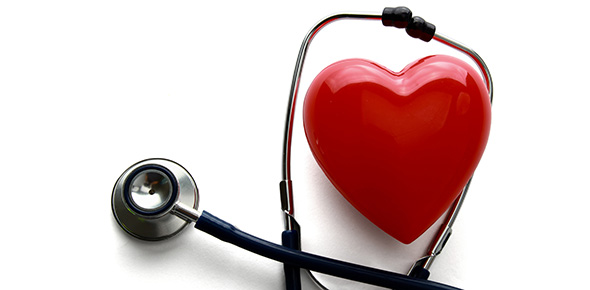# Cardiac Math Formulas (A&p2 @ Gwinnett Tech)

20 Questions | Attempts: 1215
ShareSettingsA method for drilling/practicing the formulas for Cardiac Math. .

• 1.
If a patient has a blood pressure of 120/80, what would the Mean Arterial Pressure (MAP) be? (Note, MAP = DP+(PP/3), PP=SP-DP)
• A.

120

• B.

80

• C.

93.33

• D.

133.33

• 2.
If a patient has a blood pressure of 160/110, what would the Mean Arterial Pressure (MAP) be?  (Note, MAP = DP+(PP/3), PP=SP-DP)
• A.

126.67

• B.

176.67

• C.

160

• D.

110

• 3.
If a patient has a blood pressure of 97/60, what would the Mean Arterial Pressure (MAP) be?  (Note, MAP = DP+(PP/3), PP=SP-DP)
• A.

60

• B.

109.33

• C.

97

• D.

72.33

• 4.
A patient has a heart rate of 65bpm and a stroke volume(SV) of 70ml, what would the cardiac output (CO)? (Note: CO=SV*HR, 1000ml = 1L)
• A.

4550L/minute

• B.

4.55L/minute

• C.

45.50L/minute

• D.

5.2L/minute

• 5.
A patient has a heart rate of 78bpm and a stroke volume(SV) of 80ml, what would the cardiac output (CO)? (Note: CO=SV*HR, 1000ml = 1L)
• A.

5.92L/minute

• B.

7.8L/minute

• C.

6.24L/minute

• D.

1.22L/minute

• 6.
A patient has a heart rate of 45bpm and a stroke volume(SV) of 59ml, what would the cardiac output (CO)? (Note: CO=SV*HR, 1000ml = 1L)
• A.

2.66L/minute

• B.

2650L/minute

• C.

6.22L/minute

• D.

1.33L/minute

• 7.
A patient has a blood pressure of 180/120, a heart rate of 87bmp, a stroke volume(SV) of 70ml and an ESV(End Systolic Volume) of 90ml. What is the EDV(End Diastolic Volume)? (Note: EDV=ESV+SV)
• A.

160ml

• B.

20ml

• C.

60ml

• D.

157ml

• 8.
A patient has a stroke volume(SV) of 47ml and an ESV(End Systolic Volume) of 72ml. What is the EDV(End Diastolic Volume)? (Note: EDV=ESV+SV)
• A.

72ml

• B.

338ml

• C.

119ml

• D.

25ml

• 9.
A patient has a blood pressure of 120/80, a stroke volume(SV) of 70ml and an EDV(End Diastolic Volume) of 140ml. What is the ESV(End Systolic Volume)? (Note: EDV=ESV+SV, so ESV = ?)
• A.

150ml

• B.

140ml

• C.

70ml

• D.

1.2L

• 10.
A patient has a stroke volume(SV) of 66ml and an EDV(End Diastolic Volume) of 116ml. What is the ESV(End Systolic Volume)? (Note: EDV=ESV+SV, so ESV = ?)
• A.

66ml

• B.

50ml

• C.

182ml

• D.

1.8L

• 11.
If the EDV is 100ml and the SV is 70ml, ESV will be ?
• A.

30ml

• B.

170ml

• C.

.7ml

• D.

1.43ml

• 12.
Stroke Volume X Heart Rate =
• A.

Cardiac Output

• B.

Cardiac Cycle

• C.

Blood Pressure

• D.

Pulse Pressure

• 13.
Length of the cardiac cycle if the heart rate is 70
• A.

0.3seconds

• B.

0.7seconds

• C.

1.2seconds

• D.

0.8seconds

• 14.
Length of the cardiac cycle if the heart rate is 95
• A.

0.63seconds

• B.

0.95seconds

• C.

0.70seconds

• D.

1.8seconds

• 15.
How long would the diastole portion of the cardiac cycle be if the heart rate is 78bpm? (Note, Systole is always .3seconds)
• A.

1.3seconds

• B.

0.47seconds

• C.

0.3seconds

• D.

0.71seconds

• 16.
EDV - SV is what
• A.

ESV

• B.

PP

• C.

CC

• D.

CO

• 17.
EDV is found by adding the SV to what?
• A.

CC

• B.

CO

• C.

EF

• D.

ESV

• 18.
DP+ (PP/3) is equal to what?
• A.

EDV

• B.

SV

• C.

MAP

• D.

CC

• 19.
A patient with a stroke volume of 80ml, an EDV of 170ml has an Ejection Fraction of?
• A.

47%

• B.

90%

• C.

72%

• D.

50%

• 20.
A patient with a stroke volume of 68ml, an EDV of 118ml has an Ejection Fraction of?
• A.

72%

• B.

58%

• C.

12%

• D.

WHAT?!

## Related TopicsBack to top
×

Wait!
Here's an interesting quiz for you.